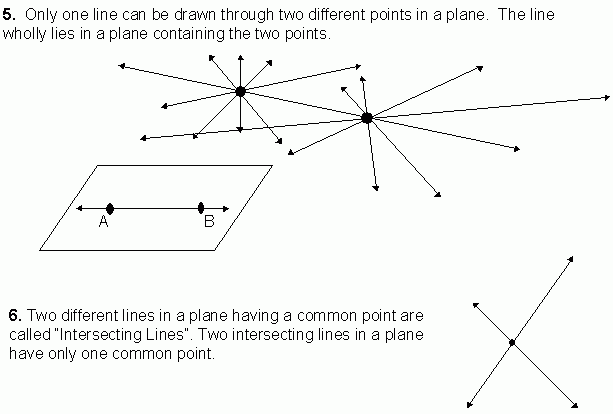Email us to get an instant 20% discount on highly effective K-12 Math & English kwizNET Programs!

#### Online Quiz (WorksheetABCD)

Questions Per Quiz = 2 4 6 8 10

### MEAP Preparation - Grade 7 Mathematics4.29 Basic Geometrical Concepts - Parallel Lines, Collinear Points and PlaneFor videos and additional resources on this topic, please see: MyndBook.com/go.php?to=207_885 Directions: Answer the following questions. Also on a sheet of paper draw a collinear points, non-collinear points and parallel and intersecting lines.
 Q 1: Number of lines that can be drawn through three non-collinear points, taking two points at a time is _____.threeonetwo Q 2: The surface of a box, the cover page of a book etc., are examples of parts of _____.pointsplaneslines Q 3: Number of lines that can be drawn through collinear points is _____.twooneinfinity Q 4: Six points are on a paper such that not any three of them are collinear. Then number of lines that can be drawn joining two points at a time is _____.51510 Q 5: A plane is a set of _______.pointsplaneslines Q 6: The flat surface of a white board gives an idea of a _____.lineplanepoint Question 7: This question is available to subscribers only! Question 8: This question is available to subscribers only!